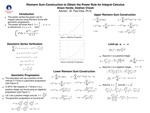## Stander Symposium Projects

#### Presenter(s)

Siobhan R. Chawk, Alison L. Hardie

This poster reflects research conducted as part of a course project designed to give students experience in the research process. Course: MTH 168

#### Description

The power rule for integration is highly studied and applied in calculus. Integral calculus is widely used in various fields outside of mathematics including science and engineering. There are multiple methods of solving integrals and this poster will demonstrate a proof of the power rule for integration through the construction of a Riemann sum. The integral that this poster will focus on is the integral of x to the power of k, in which k is assumed to be positive and rational. The sum constructed in this poster utilizes a geometric partition. Following the construction of the lower Riemann sum the limit as n approaches infinity is taken to eliminate the dependence on n. An upper Riemann sum is constructed, and the sandwich theorem is applied to the two sums to complete the proof of the original statement.

4-22-2021

Course Project

Paul W. Eloe

Mathematics

#### Keywords

Stander Symposium project, College of Arts and SciencesCOinS